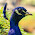# Bash Scripting - 'until' Loop

Bash 'until' loop - We have been discussing loops in shell scripting and so far, we have covered two of its kinds - for loop and while loop, in two of our recent articles. In this article, we will be covering another type of a loop - `until` loop. If you have already read about `while` in bash scripting, you will find that `until` loop behaves exactly opposite to that of `while` loop. How, lets see it.

### The 'until' Loop

In `until` loop, just the same as `while` loop, we have a condition, which is checked after every iteration. But the difference is here, which makes `until` loop's behavior exactly opposite to the `while` loop. In `until` loop,as long as the condition stays False, the block of code keeps executing, thus forming a loop. As soon as the condition becomes True, the loop is terminated.

Syntax:

```until [ Condition ]
do

-- Block of Commands --

done
```

Example:

```#!/bin/bash

n=1

until [ \$n -gt 5 ]
do
echo "This is Iteration No. \$n"
n=\$( expr \$n + 1 )
done
```

In above code, we have `n=1` before we enter the loop. When we enter the loop, the condition is checked whether `n <= 5`, which is False, and we enter the loop. Now, `echo` statement displays current value of `n` which is 1. Then, `n` is incremented by 1 (Please check our article on Arithmetic Operations for more details about `expr`). When the block ends, we have `n=2` and the control goes back to `until`, where the condition is checked again. Condition follows, `echo` prints the value of `n` and increments its value by 1. So, after 5 iterations, we would have `echo` statement executed 5 times and with `n` incremented to value 6. At this point, control goes to `until`, condition is checked and evaluated to be True. Hence the loop exits here. In the end, we have below result in the output.

```This is Iteration No. 1
This is Iteration No. 2
This is Iteration No. 3
This is Iteration No. 4
This is Iteration No. 5
```

### An Infinite 'until' Loop

An infinite `until` loop is the one, which never ends. So, for this, you can mention an expression that evaluates to False. The condition always being False, the block of code will keep executing infinitely, without exiting.

Example:

```#!/bin/bash

until [ 5 -ne 5 ]
do
echo "You are in an Infinite Loop. Press CTRL + C to Exit.."
done
```

### 'break' Statement

The `break` statements are used in the loops to exit from the loop prematurely, based on certain condition (Please take look over our article on Bash Conditionals). If the condition follows, `break` statement is executed, which then prevents further iterations from happening and exits from loop.

Syntax:

```until [ condition ]
do
-- Some Commands --

if [ condition ]
then
break
fi

-- More commands --

done
```

Example:

```#!/bin/bash

until [ 5 -gt 5 ]
do
echo "Enter a single-digit number :"

if [ \$n -gt 9 ]
then
echo "Wrong Entry! Program will terminate now.."
break
fi

echo "Good!"
done
```

Output:

```Enter a single-digit number :
1
Good!
Enter a single-digit number :
9
Good!
Enter a single-digit number :
10
Wrong Entry! Program will terminate now..
```

### 'continue' Statement

The continue statement in a loop, when certain condition becomes true, skips all the subsequent statements, coming after it, and continues with the next iteration of the loop. So, when a `continue` statement is reached, further portion of code is skipped from execution and next iteration is started.

Syntax:

```until [ condition ]
do
-- Some Commands --

if [ condition ]
then
continue
fi

-- More commands --

done
```

Example:

We take an example of printing all even numbers less than 20. In this case, we check whether a number is perfectly divisible by 2, using mod operator. If it is not, its clearly an odd number, so we prefer not to print it and skip further processing. For this, we introduce a `continue` statement in this condition, to prevent odd numbers from printing.

```#!/bin/bash

i=0
until [ \$i -gt 20 ]
do
i=\$(expr \$i + 1)
j=\$(expr \$i % 2)

if [ \$j -ne 0 ]
then
continue
fi

echo "\$i"
done
```

Output:

```2
4
6
8
10
12
14
16
18
20
```

Exercise: Sum of squares of first 'N' natural numbers

```#!/bin/bash

echo "Enter the Last Number"

i=1
sum=0

until [ \$i -gt \$n ]
do
j=\$(expr \$i \* \$i)
sum=\$(expr \$sum + \$j)
i=\$(expr \$i + 1)
done

echo "Sum of Squares of First \$n Natural Numbers = \$sum"
```

Result:

```Enter the Last Number
6
Sum of Squares of First 6 Natural Numbers = 91
```

With this, we have come to an end of this discussion on bash `until` loops. In this article, we've learned how `until` are constructed and how the control flow works. We have also revised how `break` and `continue` statements work in until loops. Please share your views and feedback in the comments section below and stay tuned. Thank you.

#### 1 comment:

1.